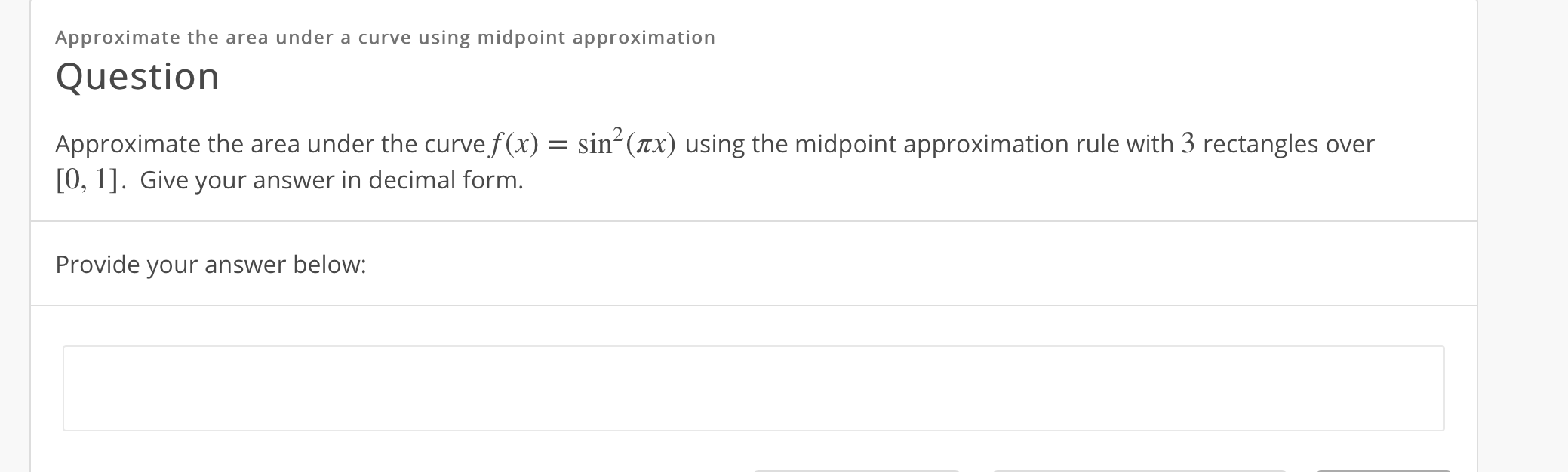# Approximate the area under a curve using midpoint approximationQuestionApproximate the area under the curve f(x) = sin- (ax) using the midpoint approximation rule with 3 rectangles over[0, 1]. Give your answer in decimal form.Provide your answer below:

Question
29 viewshelp_outlineImage TranscriptioncloseApproximate the area under a curve using midpoint approximation Question Approximate the area under the curve f(x) = sin- (ax) using the midpoint approximation rule with 3 rectangles over [0, 1]. Give your answer in decimal form. Provide your answer below: fullscreen
check_circle

star
star
star
star
star
1 Rating
Step 1

Given:

Step 2

Formula used:

Step 3

### Want to see the full answer?

See Solution

#### Want to see this answer and more?

Solutions are written by subject experts who are available 24/7. Questions are typically answered within 1 hour.*

See Solution
*Response times may vary by subject and question.
Tagged in

### Calculus